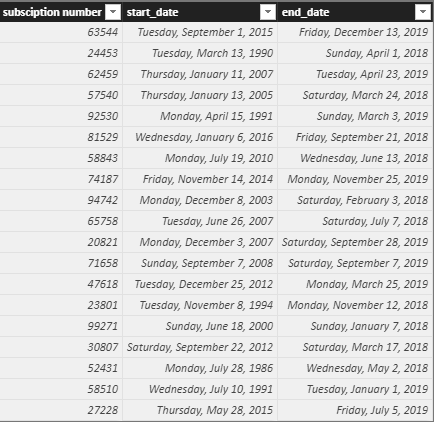• Home  /

# Calculate the Time between Two Dates in Power BI

Calculating the time between two dates is a rather simple task. You will just need a starting point and an ending point that has been formatted as a date or time. We can leverage Power BI native functions to get us the data we are looking for.  We will create columns that result in the number of days, months, weeks, minutes, seconds, or years between the two points. Calculating the time difference follows the same process.  In this example, we will be evaluating the length of subscriptions by account.  Let’s take a look at the data. Master Power BI with Discount Courses at EnterpriseDNAThe table has the subscription number and a start and end date.  We would like to create new columns to create the number of days, months, and years between dates.  Follow the instructions below.  We will be using the DATEDIFF function. This function exists in both Excel and SQL so it can be used any many circumstances to calculate the time between a starting point and an ending point. Let’s take a look at the formula

`DATEDIF(date1,date2,interval)`

The interval part of the equation can be a day, month, year, quarter, minute and second.  So don’t limit yourself to the example below.

1. Click on New Columns in the top pain in the Modeling section.2.  Enter the following formula into the formula bar, you can choose the name that is most appropriate. For this example, I going to use # of days for my heading.

`# of days = DATEDIFF('Table'[start_date],'Table'[end_date],DAY)`Now let’s create a column for the # of months and the # of years.

`# of months = DATEDIFF('Table'[start_date],'Table'[end_date],MONTH)`
`# of years = DATEDIFF('Table'[start_date],'Table'[end_date],YEAR)`

Remember you are not limited to just days, you can also get minutes in seconds by just changing the intervals.</pre>

### Other Articles

Create Python Visuals in Power BI